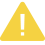# Python 并发编程之线程队列

【摘要】 线程队列的使用级说明知识。

### 线程队列

queue队列 ：使用import queue，用法与进程Queue一样

queue is especially useful in threaded programming when information must be exchanged safely between multiple threads.

• class `queue.``Queue`(maxsize=0) #先进先出

```import queue

q=queue.Queue()
q.put('first')
q.put('second')
q.put('third')
print(q.get())
print(q.get())
print(q.get())
'''

first
second
third
'''```

class `queue.``LifoQueue`(maxsize=0) #last in fisrt out

```import queue

q=queue.LifoQueue()
q.put('first')
q.put('second')
q.put('third')
print(q.get())
print(q.get())
print(q.get())
'''

third
second
first
'''```

```import queue

q=queue.PriorityQueue()
#put进入一个元组,元组的第一个元素是优先级(通常是数字,也可以是非数字之间的比较),数字越小优先级越高
q.put((20,'a'))
q.put((10,'b'))
q.put((30,'c'))
print(q.get())
print(q.get())
print(q.get())
'''

(10, 'b')
(20, 'a')
(30, 'c')
'''```

```Constructor for a priority queue. maxsize is an integer that sets the upperbound limit on the number of items that can be placed in the queue.
Insertion will block once this size has been reached, until queue items are consumed. If maxsize is less than or equal to zero,
the queue size is infinite.
The lowest valued entries are retrieved first (the lowest valued entry is the one returned by sorted(list(entries))).
A typical pattern for entries is a tuple in the form: (priority_number, data).
exception queue.Empty
Exception raised when non-blocking get() (or get_nowait()) is called on a Queue object which is empty.
exception queue.Full
Exception raised when non-blocking put() (or put_nowait()) is called on a Queue object which is full.
Queue.qsize()
Queue.empty() #return True if empty
Queue.full() # return True if full
Queue.put(item, block=True, timeout=None)
Put item into the queue. If optional args block is true and timeout is None (the default), block if necessary until a free slot is available.
If timeout is a positive number, it blocks at most timeout seconds and raises the Full exception if no free slot was available within that time.
Otherwise (block is false), put an item on the queue if a free slot is immediately available,
else raise the Full exception (timeout is ignored in that case).
Queue.put_nowait(item)
Equivalent to put(item, False).
Queue.get(block=True, timeout=None)
Remove and return an item from the queue. If optional args block is true and timeout is None (the default),
block if necessary until an item is available. If timeout is a positive number,
it blocks at most timeout seconds and raises the Empty exception if no item was available within that time. Otherwise (block is false),
return an item if one is immediately available, else raise the Empty exception (timeout is ignored in that case).
Queue.get_nowait()
Equivalent to get(False).
Two methods are offered to support tracking whether enqueued tasks have been fully processed by daemon consumer threads.
Indicate that a formerly enqueued task is complete. Used by queue consumer threads. For each get() used to fetch a task,
a subsequent call to task_done() tells the queue that the processing on the task is complete.
If a join() is currently blocking, it will resume when all items have been processed (meaning that a task_done() call was received
for every item that had been put() into the queue).
Raises a ValueError if called more times than there were items placed in the queue.
Queue.join() block直到queue被消费完毕```

【版权声明】本文为华为云社区用户原创内容，转载时必须标注文章的来源（华为云社区），文章链接，文章作者等基本信息，否则作者和本社区有权追究责任。如果您发现本社区中有涉嫌抄袭的内容，欢迎发送邮件至：huaweicloud.bbs@huawei.com进行举报，并提供相关证据，一经查实，本社区将立刻删除涉嫌侵权内容。

### 评论 (0)

###### 温馨提示